# Spot 2D Shapes on 3D Solids

In this worksheet, students identify 2D shapes found on common 3D solids.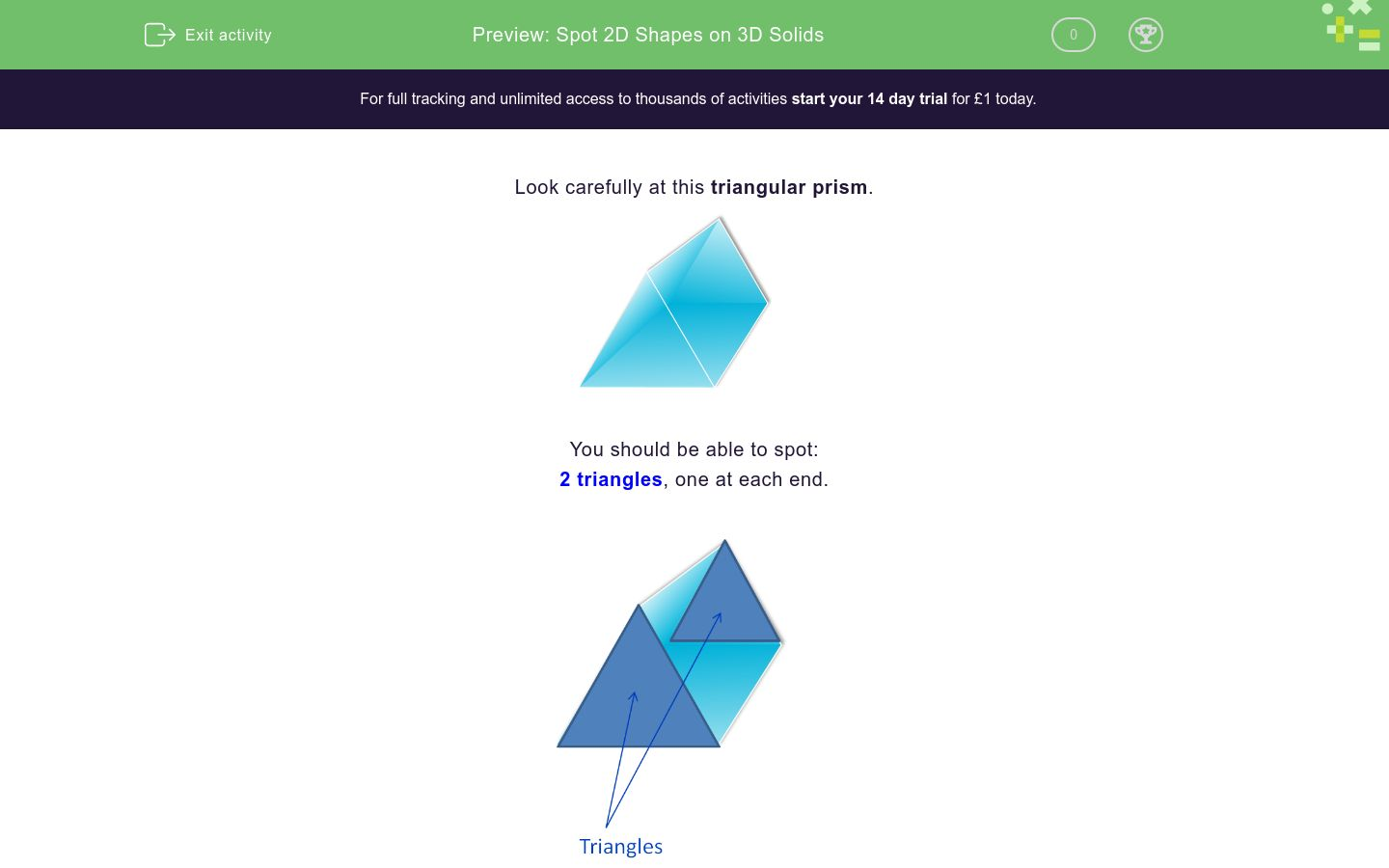Key stage:  KS 1

Curriculum topic:   Geometry: Properties of Shapes

Curriculum subtopic:   Identify 2D with 3D Shapes

Difficulty level:### QUESTION 1 of 10

Look carefully at this triangular prism.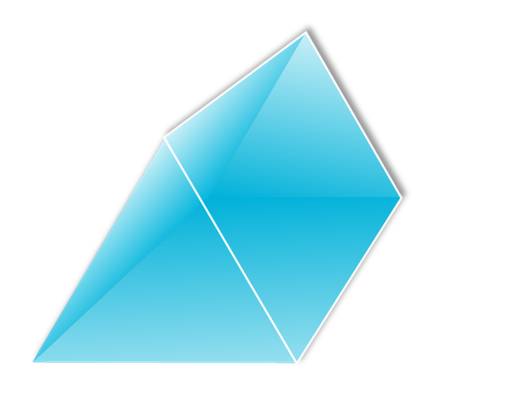You should be able to spot:

2 triangles, one at each end.and 3 rectangles between the triangles.Here is a cube.

What shapes can you see on its faces?triangles

squares

circles

pentagons

Here is a cuboid.

What shapes can you see on its faces?squares

triangles

circles

rectangles

Here is a cylinder.

What flat shapes can you see on its faces?circles

rectangles

squares

triangles

Here is a sphere.

What flat shapes can you see on its faces?circles

triangles

squares

rectangles

none of these

Here is a cone.

What flat shapes can be found on its faces?circle

rectangle

square

triangle

Here is a prism.

Which shapes can be found on its faces?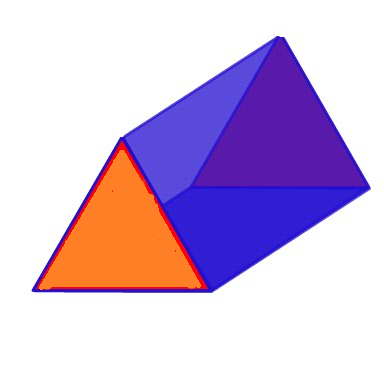circles

rectangles

squares

triangles

Here is a cylinder.

Which shapes can be found on its faces?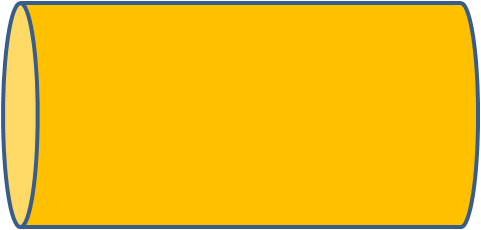circles

rectangles

squares

triangles

Here is a pyramid.

Which shapes can be found on its faces?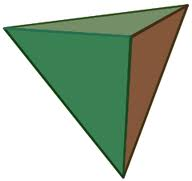circles

rectangles

squares

triangles

Here is a pyramid.

Which shapes can be found on its faces?circles

rectangles

square

triangles

Here is an octahedron.

Which shapes can be found on its faces?circles

rectangles

squares

triangles

• Question 1

Here is a cube.

What shapes can you see on its faces?squares
EDDIE SAYS
A cube is made of 6 squares.
• Question 2

Here is a cuboid.

What shapes can you see on its faces?rectangles
EDDIE SAYS
This cuboid is made of 6 rectangles.
• Question 3

Here is a cylinder.

What flat shapes can you see on its faces?circles
EDDIE SAYS
A cylinder has a circle at the top and at its base.
• Question 4

Here is a sphere.

What flat shapes can you see on its faces?none of these
EDDIE SAYS
There are no flat shapes on a sphere.
• Question 5

Here is a cone.

What flat shapes can be found on its faces?circle
EDDIE SAYS
A cone has a circle at its base.
• Question 6

Here is a prism.

Which shapes can be found on its faces?rectangles
triangles
EDDIE SAYS
It has a triangle at each end and rectangles between.
• Question 7

Here is a cylinder.

Which shapes can be found on its faces?circles
EDDIE SAYS
It has a circle at each end.
• Question 8

Here is a pyramid.

Which shapes can be found on its faces?triangles
EDDIE SAYS
It is made of 4 triangles.
• Question 9

Here is a pyramid.

Which shapes can be found on its faces?square
triangles
EDDIE SAYS
It is made of 4 triangles and a square base.
• Question 10

Here is an octahedron.

Which shapes can be found on its faces?triangles
EDDIE SAYS
It is made of 8 triangles.
The square is inside the shape and so is not one of its faces.
---- OR ----

Sign up for a £1 trial so you can track and measure your child's progress on this activity.

### What is EdPlace?

We're your National Curriculum aligned online education content provider helping each child succeed in English, maths and science from year 1 to GCSE. With an EdPlace account you’ll be able to track and measure progress, helping each child achieve their best. We build confidence and attainment by personalising each child’s learning at a level that suits them.

Get started